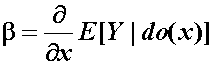# Causal Analysis in Theory and Practice

## January 1, 2001

### On the Causal Interpretation of Path Coefficients

Filed under: Path Coefficient — moderator @ 12:00 am

From Les Hayduk, University of Alberta:

Les Hayduk asked whether the operational formula for path coefficient;(Eq. 5.24)(Causality, page 161, Eq. (5.24)) is a calculation formula or purely an interpretation formula.

1. Like all models in science, a structural equation model (SEM) is interpreted as a mapping between physical operations in the real world (observations, interventions, etc.) and their representative mathematical operations on the model.&nbsp; The physical operation, denoted by do(x), corresponds to grabbing variable X, setting its value at X=x, holding it at that setting and observing what happens to other variables in the world.&nbsp; The mathematical operation that represents this intervention corresponds to removing the equation for X from the model, replacing it by the equation X=x, and computing the behavior of other variables in the newly created model. The claims made by an SEM model are encapsulated in expressions of the type
E(Y|do(x)) = 0.48 x + 0.10or
E(Y|do(x+1,z)) – E(Y|do(x,z)) = 0.65and these correspond of course to predictions about what we should observe in the real world if we were to execute those interventions and measure those expectations.&nbsp; Thus, the operational formula for b (Causality, page 161),&nbsp;&nbsp;&nbsp;&nbsp;&nbsp;&nbsp;&nbsp;&nbsp;&nbsp;&nbsp;&nbsp;&nbsp;&nbsp;&nbsp;&nbsp;&nbsp;&nbsp;&nbsp;&nbsp;&nbsp; (Eq. 5.24)

is both interpretational and computational. As an interpretation of b, the formula makes predictions about empirical observations in the world and, as a computational device, it permits the computation of b from a (fully specified) model through the do(x) operator.&nbsp; In addition, it also permits the computation of b in a partially specified model, by relating it to other model parameters, especially those that can be estimated through experimental and nonexperimental data. This often leads to the derivation of statistical estimands for b that are based strictly on covariances of observed variables.

Best wishes,
========Judea Pearl

Comment by judea — January 1, 2001 @ 12:00 pm

2. Google will reportedly release one phone with a 5.2-inch screen and a separate with a 5.7-inch display. To put those sizes in perspective, a 5.2-inch phone would be slightly larger than the Samsung Galaxy S6, which has a 5.1- inch screen.

Comment by Christian Dior iPhone 6 + Case — May 28, 2015 @ 12:34 am